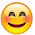# Lesson Planning of Picture Graphs

Lesson Planning of Picture Graphs

Subject Mathematics

Students` Learning Outcomes

• Read and interpret picture graphs

Information for Teachers

• Graphs are pictures that help us understand information in the form of figures. The information is called data. There are many kinds of graphs, each having special parts.• A picture graph (also known as a pictograph) is one of the simple types of graph.
• Picture graphs convey information by using relevant pictures as symbols to represent information.
•• While teaching this lesson, the teacher should also consult textbook at all steps wherein and whenever it is applicable.

Material / Resources

Writing board, chalk/marker, duster, picture graph of fruit, picture graph of balloon, picture graph of parents, sheets of paper, textbook

Introduction

• Tell the following story
• Ahmad bought different types of fruit from the market yesterday.
• Display the following picture chart to the students or make the same chart on the board by drawing pictures of the each fruit to show the type and quantity of each fruit bought by Ahmad.
• Tell them that this type of chart is called picture graph because it represents information in numbers using pictures.
• Ask them to look at the chart and tell how many apples, pears, mangoes, oranges and strawberries did Ahmad but?
• How can you tell?

Development

 Activity 1 Demonstrate to the students that how they will interpret a picture graph. Show the above picture graph to the students and ask the following question: How many apples are there in this graph? Which fruit is least in number? How many more mangoes are there than orange?

 Activity 2 Fatima bought following balloons of different colours. Six red balloons Four blue balloons Five yellow balloons Six green balloons Show the picture graph of Fatima`s balloons to the students.Ask the students, what this graph shows? After taking the response of students, explain the above picture graph to them and ask to complete the following; Fatima has __________ red balloons. She has ____________green balloons. She has ___________ more red balloons than blue balloons. She has same number of _____ and _______ balloon. She has ___________balloons, altogether.

 Activity 3 Display the following graph in the class. Tell the students that this graph shows the number of parents who visited the ABC school in a week.Key: symbol  stands for 2 parents =  Tell the students that in this graph symbol is given. Ask students to answer the following questions: How many parents visited the school of Friday? (Expected answer would be as; 14) On which day least number of parents visited the school? (Expected answer would be as; Wednesday) On which day maximum number of parents visited the school? (Expected answer would be as; Saturday) On Sunday how many parents visited the school? (Expected answer would be as; 00)

Sum up / Conclusion

• Graphs are pictures that help us understand information in the form of figures. The information is called data.
• A picture graph (also known as a pictograph) is one of the simplest types of graph. Picture graphs convey information by using relevant pictures as symbols to represent information.

Assessment

• Show the picture graph of fruit to the students and ask the following questions;
• How many fruit are there altogether?
• Which fruit is less in number, strawberry or orange?
• Which fruit is more in number?

• Draw this graph in your copy and write answer the following questions;

No. of balloons =

No. of apples =

No. of oranges =

No. of mangoes =

Which is maximum in number =

Which is minimum in number/

Ask the students to solve the questions given in the text book.